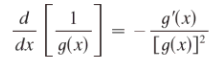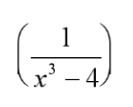# Reciprocal Rule: Definition, Examples

In calculus, the reciprocal rule can mean one of two things:

In basic math, there is also a reciprocal rule for division, where the basic idea is to invert the divisor and multiply. Although not the same thing, it’s a similar idea (at one step in the process you invert the denominator). There are reciprocal rules in various other fields, like photography and trace theory, but they have no relation to the math-based rules outlined here.

## 1. Reciprocal Rule Formula (Derivatives)

This rule for finding the derivative of a reciprocal function is almost the same as the quotient rule. However, you can only use the reciprocal rule if your numerator is a constant.

Assuming that a function is differentiable, you can find the derivative with the following formula (Stewart, 2009):The basic steps are:

1. Find the derivative of the denominator and place it as the numerator (i.e. on top) of a new fraction,
2. Square the denominator and place it in the denominator (on the bottom) of the new fraction.
3. Add a negative sign to the new fraction.

This is a very useful rule for finding derivatives of functions that have that fractional format. However, you will have to find the derivative of the denominator, so you may have to use other rules for derivatives there (like the power rule or the chain rule). Note: Make sure your denominator isn’t equal to zero, otherwise this rule will not work.

## Reciprocal Rule Example (Derivatives)

Note: This example uses the power rule to work the formula. If you’re unfamiliar with that rule, watch the short video below the answer for how it works.

Example question: Find the derivative of the following function, using the reciprocal rule:1. The derivative of x3 – 4 is 3x2, so:2. Square the function in the denominator and place it in the denominator of the new fraction.3. Don’t forget to add the negative sign!

## Reciprocal Rule (Limits)

The reciprocal rule for limits states that the limit of the reciprocal of a function equals the reciprocal of the limit of the function as x approaches a . As a formula, that’s:If it looks (and sounds) complicated, it really isn’t. It uses the same common sense as saying “99.9999 is close to 100.” That said, the proof is “tedious” (Taalman, 2013), so you won’t usually come across it in most mainstream textbooks other than a brief mention.

## Reciprocal Function

A reciprocal function is the multiplicative inverse of a “regular” function: a function that, when multiplied by the original function, results in the constant 1.is the reciprocal function.

## Confusion in Terminology

Although the reciprocal is the multiplicative inverse of a function, it is important to realize that this may not be the same as the ‘inverse’ of that function. While the reciprocal is the function that, when multiplied by the original, equals 1, the inverse of a function is one which when taken as a composite with the original function, becomes x.

The inverse is usually designated by f-1(x). The multiplicative inverse can be designated by [f(x)]-1.

For an inverse, f-1(x), f( f-1(x) ) = x and f-1( f(x) ) = x. For a multiplicative inverse (reciprocal) g(x), f(x) · g(x) = 1.

## Examples of Reciprocal Functions

The reciprocal of the function f(x) = x is just g(x)= 1/x. The image below shows both functions, graphed on the same graph. The original function is in blue, while the reciprocal is in red. Note that in this case the reciprocal, or multiplicative inverse, is the same as the inverse f-1(x).The reciprocal of the function f(x) = x + 5 is g(x) = 1/ (x + 5). Note that in this case the reciprocal (multiplicative inverse) is different than the inverse f-1(x). For this function, f-1(x) = x – 5. Below all three functions are graphed; the original in red, the reciprocal in blue and the inverse in green.## References

Blackie’s Dictionary of Mathematics.